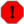## ptablew

ptablew — Change the contents of existing function tables of any length.

## Description

This opcode operates on existing function tables, changing their contents. ptablew is for writing at k- or at a-rates, with the table number being specified at init time. Using ptablew with i-rate signal and index values is allowed, but the specified data will always be written to the function table at k-rate, not during the initialization pass. The valid combinations of variable types are shown by the first letter of the variable names.

## Syntax

`ptablew asig, andx, ifn [, ixmode] [, ixoff] [, iwgmode]`
`ptablew isig, indx, ifn [, ixmode] [, ixoff] [, iwgmode]`
`ptablew ksig, kndx, ifn [, ixmode] [, ixoff] [, iwgmode]`

## Initialization

asig, isig, ksig -- The value to be written into the table.

andx, indx, kndx -- Index into table, either a positive number range matching the table length (ixmode = 0) or a 0 to 1 range (ixmode != 0)

ifn -- Table number. Must be >= 1. Floats are rounded down to an integer. If a table number does not point to a valid table, or the table has not yet been loaded (GEN01) then an error will result and the instrument will be de-activated.

ixmode (optional, default=0) -- index mode.

• 0 = xndx and ixoff ranges match the length of the table.

• !=0 = xndx and ixoff have a 0 to 1 range.

ixoff (optional, default=0) -- index offset.

• 0 = Total index is controlled directly by xndx, i.e. the indexing starts from the start of the table.

• !=0 = Start indexing from somewhere else in the table. Value must be positive and less than the table length (ixmode = 0) or less than 1 (ixmode != 0).

iwgmode (optional, default=0) -- Wrap and guardpoint mode.

• 0 = Limit mode.

• 1 = Wrap mode.

• 2 = Guardpoint mode.

## Performance

### Limit mode (0)

Limit the total index (ndx + ixoff) to between 0 and the guard point. For a table of length 5, this means that locations 0 to 3 and location 4 (the guard point) can be written. A negative total index writes to location 0.

### Wrap mode (1)

Wrap total index value into locations 0 to E, where E is one less than the table length. For example, wrap into a 0 to 3 range - so that total index 6 writes to location 2.

### Guardpoint mode (2)

The guardpoint is written at the same time as location 0 is written - with the same value.

This facilitates writing to tables which are intended to be read with interpolation for producing smooth cyclic waveforms. In addition, before it is used, the total index is incremented by half the range between one location and the next, before being rounded down to the integer address of a table location.

Normally (igwmode = 0 or 1) for a table of length 5 - which has locations 0 to 3 as the main table and location 4 as the guard point, a total index in the range of 0 to 0.999 will write to location 0. ("0.999" means just less than 1.0.) 1.0 to 1.999 will write to location 1 etc. A similar pattern holds for all total indexes 0 to 4.999 (igwmode = 0) or to 3.999 (igwmode = 1). igwmode = 0 enables locations 0 to 4 to be written - with the guardpoint (4) being written with a potentially different value from location 0.

With a table of length 5 and the iwgmode = 2, then when the total index is in the range 0 to 0.499, it will write to locations 0 and 4. Range 0.5 to 1.499 will write to location 1 etc. 3.5 to 4.0 will also write to locations 0 and 4.

This way, the writing operation most closely approximates the results of interpolated reading. Guard point mode should only be used with tables that have a guardpoint.

Guardpoint mode is accomplished by adding 0.5 to the total index, rounding to the next lowest integer, wrapping it modulo the factor of two which is one less than the table length, writing the table (locations 0 to 3 in our example) and then writing to the guard point if index = 0.

ptablew has no output value. The last three parameters are optional and have default values of 0.

### Caution with k-rate table numbers

At k-rate or a-rate, if a table number of < 1 is given, or the table number points to a non-existent table, or to one which has a length of 0 (it is to be loaded from a file later) then an error will result and the instrument will be deactivated. kfn and afn must be initialized at the appropriate rate using init. Attempting to load an i-rate value into kfn or afn will result in an error.

WarningNote that ptablew is always a k-rate opcode. This means that even its i-rate version runs at k-rate and will write the value of the i-rate variable. For this reason, the following code will not work as expected: ``` giFt ftgen 1, 0, 8, 2, 0 instr 1 indx = 0 ptablew 10, indx, giFt ival tab_i indx, giFt print ival endin i 1 0 1 ``` Although it may seem this program should print a 10 to the console. It will print 0, because tab_i will read the value at the initialization of the note, before the first performance pass, when ptablew writes its value.